# Lesson 3: Price Data for Two Markets

This lesson is a continuation of Lesson 1: Maximizing Profit and Market Saturation and Lesson 2: Price Data and Trendlines,  where we considered the profit from one market with price per unit function that depended only on the sales in a single market and considered how to find the parameters of that linear function. In this lesson we will consider the two market problem, where the prices per unit in each market depend on sales in both markets.The math model for the price data will be linear in each variable for the sales. However, the resulting algebraic problem is a little more difficult, and so, we will use the Excel command called LINEST for linear estimation of the price data. We will indicate how this linear price function of the two sales variables can be used to determine the maximum profit.

# The Mathematical Model

Since we have two markets, we need two variables. Let's use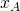to represent the number of units sold in market A, and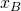to represent the number of units sold in market B. We'll assume, as we did in Lesson 1, that the price in each market responds to our selling units by dropping in a linear way. Let's say that the initial prices and price responses are as given in the following table:

 market initial price price response in market A price response in market B A 97 -0.1 -0.05 B 83 -0.005 -0.01

That is, each unit we sell in market A drops the market price in market A by 10 cents, but the price in market B only by 5 cents (some people in market B may be willing to travel to market A to buy our goods, but not everyone will). Each unit we sell in market B will drop the market price in market A by half a cent and the market price in market B by a cent.

We encode this information in a system of linear equations: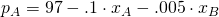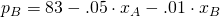Nominally, Lesson 1 was about "suits"; here we've started talking about "units". That's because none of this has to do with the specifics of suits. So we may as well work with the slightly-more-abstract setting of "units" rather than "suits". For the same reason, we may as well work with the slightly-more-abstract setting by replacing the particular numbers $97,$83, $-0.1,$-0.005, $-0.05,$-0.01 with symbols that we can replace with other values, so as to apply our model to other situations in the future.

What's a parameter and what's a variable? Both are symbols that stand for some quantity we either don't know or don't want to specify at the moment.

To some extent it's a matter of taste what you call what. I'll use the word "parameter" to mean a symbol whose value we set at the beginning of a problem and leave fixed for the whole problem. (For example, the manufacturing cost of a suit.) A variable, on the other hand, is what I'll call a symbol whose value changes within the course of a particular problem. (For example, the number of suits manufactured.)

For the remainder of this lesson, we're going to focus on the question of how we might find the values of price response parameters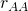,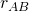,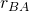, and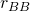given real-world sales data (which is what we did in Lesson 2 for the single-market case). Later, in Lesson 4, we'll see how to actually use such numbers to maximize the profit (good capitalists that we are).

# The Mathematical Method

We're going to use Excel to find the values of the response parameters in market A. First observe that, unlike in Lesson 2, we now have four variables at play: the independent variablesand, and the dependent variables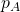and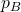. So we can't really plot the data; we'd need to do so in four-dimensional space! (Even plotting in 3-dimensional space is hard.) Nevertheless, we can use a version of the correlation-coefficient and least-squares method that we used in Lesson 2.

a video walkthrough of LINEST

Here's some price-response data:

 sales in market A sales in market B price in market A 10 5 45 12 4 44 13 3 43 15 3 39

Enter this into a new Excel worksheet.

The command we want in Excel is called "LINEST", for "linear estimation". Use it like this:

1. Highlight a 3-column by 5-row rectangle of empty cells.
2. Type "=LINEST(".3. Highlight C2:C5. Type ",".
4. Highlight A2:B5. Type ",".5. Type "TRUE,TRUE)".
6. Hit command-shift-enter.7. If you hit enter alone, you will get this result, which is different (and not what we need):Excel sometimes needs to report more than one number as output. Formulas with more than one number as output are called "array formulas". LINEST is one such formula. To execute an array formula, you must use command-shift-enter rather than plain old enter. Why? I don't know, I think it's dumb. But that's the way Excel is.

Excel has just given us a huge mess of numbers. What do do with them?

LINEST reports the best linear model for its first argument (here, the price in market A) as a function of the variables in its second argument (here, the number of units sold in markets A and B). That is, it tries to find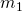,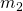, and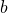so that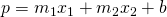is a good fit for the data. LINEST reports its results as follows:stats and stuff more stats even more stats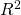even more stats dragons? # of variables - 1 sum of squares other sum of squares

Most of this stuff is irrelevant to us (so I've been a bit cheeky) but some of it is stuff we'll need.

The correlation coefficient is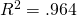, which means our data do in fact look quite linear. The coefficients are -- and here's the really annoying part -- reported exactly backwards from the order they appear in the spreadsheet. So Excel is telling us it thinks the linear model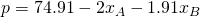is a good fit for the data we gave it.

If we wanted to know what the market price in market A would be if we sold 20 units in market A and 10 units in market B, we would evaluate# Takeaways/Deliverables

To say you've successfully completed this lesson, you should be able to do the following:

1. Use the LINEST command in Excel
2. Correctly interpret the output (parameters and correlation coefficient) of the LINEST command.
3. Use the output of LINEST to project or extrapolate a linear model from given data.

These skills are what you'll need to complete the WebAssign homework for this Lesson.